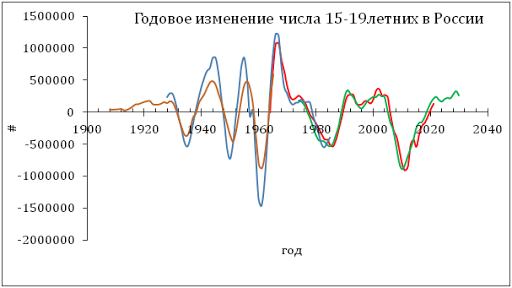## 1/28/12

### On wise monetary policy and the absence of liquidity trap in Japan

We have been following inflation in Japan since 2005 when our first paper on the Japanese economy was published. We have revisited inflation in Japan in 2010 and confirmed the predictions of deflation as expressed by the negative GDP deflator. In this blog, we also reported on deflation (both CPI and GDP deflator) several times. Here we validate our predictions of the rate of consumer price inflation (CPI) by the estimate for 2011. The Japan Bureau of Statistics has estimated the rate of CPI inflation as -0.3%.

The case of Japan is the best illustration of our concept linking inflation to the change in labour force. We assume that there was neither liquidity trap in Japan nor mistakes in monetary policy. The evolution of inflation is completely driven by the change in labour force. This is an unfortunate situation for Japan since the level of labour force can only fall in the long run due to the quickly decreasing working age population.

Previously, we carried out an estimation of empirical relationship between the change rate of labour force, dLF(t)/LF(t), and inflation, p(t).  First, we test the existence of a link between inflation and labour force. Because of the structural (measurement related?) break in the 1980s, we have chosen the period after 1982 for linear regression. By varying the lag between the labour force and inflation one can obtain the best-fit coefficients for the prediction of CPI inflation, p(t),  according to the following relationship:

p(t) = 1.43dLF(t-t0)/LF(t-t0) + 0.000         (1)

where the time lag t0=0 years; standard errors for both coefficients are shown in brackets.  Figure 1 (upper panel) depicts this best-fit case. There is no time lag between the inflation series and the labour force change series in Japan. Free term in (1), defining the level of price inflation in the absence of labour force change, is practically undistinguishable from zero.

A more precise and reliable method to compare observed and predicted inflation consists in the comparison of cumulative curves. Short-term oscillations and uncorrelated noise in data as induced by inaccurate measurements and the inevitable bias in all definitions should be smoothed out in cumulative curves. Any actual deviation between two cumulative curves persists in time if measured values are not matched by the defining relationship.

The predicted cumulative values shown in the lower panel of Figure 1 are very sensitive to the free term in (1). For Japan, the cumulative curves are characterized by complex shapes. There are periods of intensive inflation and a deflationary period. The labour force change, defining the predicted inflation curve, follows all the turns in the measured cumulative inflation.

One can conclude that relationship (1) is valid and the labour force change is the driving force of inflation. Statistically, the evolution of the overall level of consumer prices in Japan is fully defined by the change in labour force. Even the annual curves have Rsq=0.73 with all fluctuations induced by the change in labor force. The cumulative curves are characterized by Rsq=0.99. Hence, no other variable or process can affect the change in price. Otherwise, the statistically reliable link would not exist.
Effectively, this means that the Japanese monetary authorities can not create conditions for positive inflation and thus there is no liquidity trap.  The problem of deflation can be resolved only in the framework of increasing population and Figure 3 shows that the next forty years will be characterized by price deflation (both CPI adn GDP deflator) when population projections are used to extrapolated labour force.

Figure 1. Measured inflation (CPI) and that predicted from the change rate of labour force. Upper panel:  Annual curves. Lower panel: Cumulative curves between 1982 and 2009. A good agreement between the cumulative curves illustrates the predictive power of our model.

Figure 2. Scatter plot: predicted vs. measured rate of CPI inflation.

Figure 3. Inflation projection  for Japan: CPI and the GDP deflator